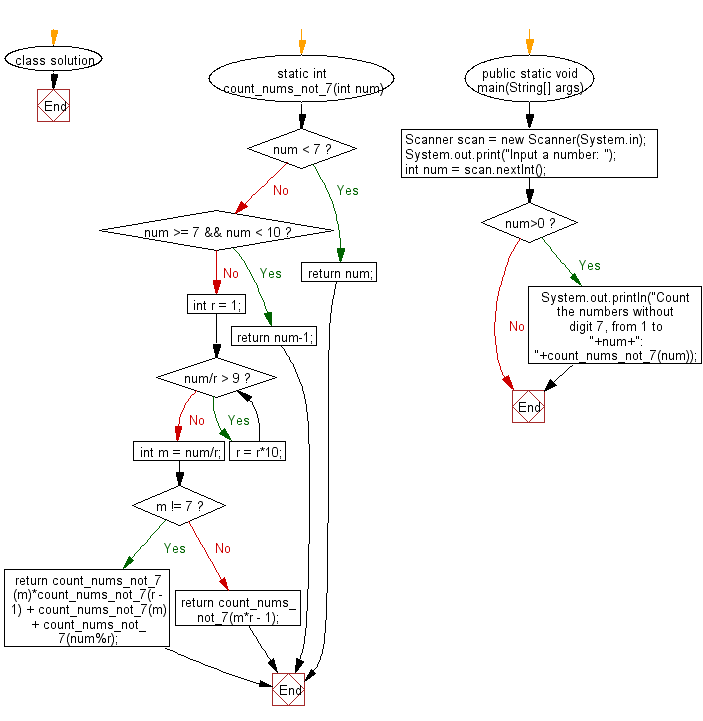﻿ Java Math Exercises: Count the numbers without digit 7, from 1 to a given number - w3resource# Java Math Exercises: Count the numbers without digit 7, from 1 to a given number

## Java Math Exercises: Exercise-18 with Solution

Write a Java program to count the numbers without digit 7, from 1 to a given number.

Sample Solution:

Java Code:

``````import java.util.*;
class solution {
static int count_nums_not_7(int num)
{
if (num < 7)
return num;
if (num >= 7 && num < 10)
return num-1;

int r = 1;
while (num/r > 9)
r = r*10;

int m = num/r;

if (m != 7)
return count_nums_not_7(m)*count_nums_not_7(r - 1) + count_nums_not_7(m) + count_nums_not_7(num%r);
else
return count_nums_not_7(m*r - 1);
}

public static void main(String[] args) {
Scanner scan = new Scanner(System.in);
System.out.print("Input a number: ");
int num = scan.nextInt();
if (num>0)
System.out.println("Count the numbers without digit 7, from 1 to "+num+": "+count_nums_not_7(num));
}
}
``````

Sample Output:

``` Input a number:  15
Count the numbers without digit 7, from 1 to 15: 14
```

Flowchart:Java Code Editor:

What is the difficulty level of this exercise?

﻿

## Java: Tips of the Day

Parsing dates:

```import java.io.*;
import java.util.*;
import java.text.*;

String s = "2001/09/23 14:39";

SimpleDateFormat formatter = new SimpleDateFormat ("yyyy/MM/dd H:mm");
Date d = formatter.parse(s, new ParsePosition(0));
```